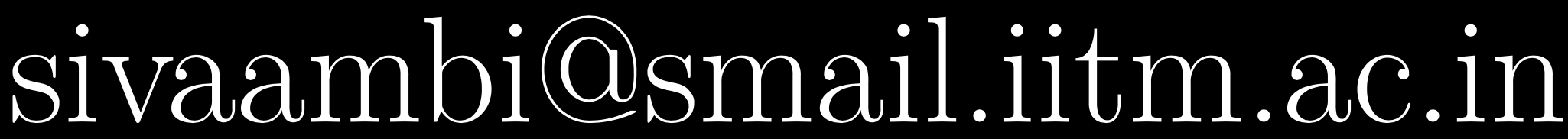# Numerical Methods and Scientific Computing, Jul-Nov 2021

### Instructor

Sivaram Ambikasaran,Evaluation Points Weekly Assignment 60 EndSem 40

### Course Logistics

• Each week there will be three pre-recorded online lectures, which can be accessed via the above link.
• There will also be assignments for a total worth of 60 points.
• There will be an endsemester worth 40 points.

### Assignments

• All assignments need to be submitted before Sunday midnight.
• There will be both written and computational part in the assignments.
• We will be relying on MATLAB/Octave for the computing part of the assignment.
• Students are strongly encouraged to typeset their solution via LaTeX/TeX. Typesetting using LaTeX/TeX will obtain 25% bonus on the score they get for the assignment.
• Students need to submit their assignments through the dropbox link provided.
• The name of the zipped file (this should contain the LaTeX/TeX source file, pdf, and MATLAB code) for submitting your assignment should be as follows: ma14m093_5.zip (or .rar or .tar), where ma14m093 is your roll number and 5 implies that you are submitting your fifth assignment.
• Only one submission per assignment. If you submit the assignment multiple times, only the first submission will be graded.
• Any copying on assignments will result in a zero on the assignment.
• We will be using JPlag to detect similarities among multiple sets of source code files.
• The grader will expect you to express your ideas clearly, legibly, and completely, often requiring complete English sentences rather than merely just a long string of equations or unconnected mathematical expressions. This means you could lose points for poorly written proofs or answers. Clear exposition is a crucial ingredient of technical communication. Clarity of thought and presentation is more important in mathematics & sciences than any other field. The only way to master exposition is by repeated practicing.

### Short Syllabus

• Floating point representation, Conditioning and Stability
• Polynomial interpolation and approximation
• Numerical Differentiation
• Numerical Integration
• Root finding
• Numerical Solution of Ordinary differential equation
• Monte Carlo methods

### Textbooks

• Numerical Methods in Scientific Computing - Volume I, by Germund Dahlquist & Ake Bjorck
• Approximation Theory and Approximation Practice, by Nick Trefethen
• Fundamentals of Engineering Numerical Analysis, by Parviz Moin
• Interpolation and Approximation by Polynomials, by George M. Phillips
• Spectral Methods in MATLAB, by Nick Trefethen
• Exploring Monte Carlo Methods, by William L. Dunn & J. Kenneth Shultis